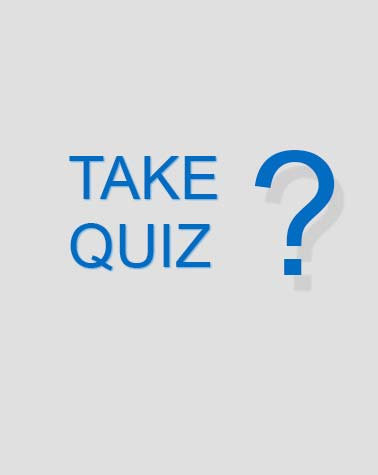# 3D156 CDC Vol. 2

124 Questions | Attempts: 53
ShareSettings• 1.
(201) The localizer equipment shelter is generally painted what colors?
• A.

International orange and white.

• B.

Olive drab and black.

• C.

Brown and tan.

• D.

Red and White.

• 2.
(201) How many assemblies support each localizer antenna?
• A.

1.

• B.

2.

• C.

3.

• D.

4.

• 3.
(202) The localizer station is designed for what type of operation?
• A.

Local control.

• B.

Remote control.

• C.

Continuous unmanned.

• D.

Intermittent unmanned.

• 4.
(202) How can the localizer station be controlled?
• A.

Locally only.

• B.

Remote only.

• C.

Locally from the site or remotely from control tower or RAPCON.

• D.

Remotely from the site or locally from the control tower or RAPCON.

• 5.
(202) What is the maximum miles per hour (mph) wind speed the localizer equipment station is designed to withstand?
• A.

50.

• B.

70.

• C.

90.

• D.

110.

• 6.
(202) How many channels do the radios in the localizer equipment station have?
• A.

One.

• B.

Two.

• C.

Three.

• D.

Four.

• 7.
(202) In megahertz (MHz), the frequency range of the transmitters in the localizer equipment station is
• A.

108 to 112.

• B.

324 to 336.

• C.

962 to 1,213.

• D.

2,250 to 2,259.

• 8.
(202) How many RF outputs are connected to the localizer antenna system for the course transmitter?
• A.

1.

• B.

2.

• C.

3.

• D.

4.

• 9.
(202) What are the modulation frequencies in the localizer course transmitter?
• A.

90,150, and 2250 Hz.

• B.

150 and 2259 Hz.

• C.

90, and 2259 Hz.

• D.

90, 150, and 1020 Hz.

• 10.
(202) What is the nominal carrier plus sideband (CSB) watt (W) output power of the course transmitter in the localizer?
• A.

10.

• B.

15.

• C.

20.

• D.

25.

• 11.
(202) What is the nominal carrier plus sideband (CSB) watt (W) output power of the clearance transmitter in the localizer?
• A.

10.

• B.

15.

• C.

20.

• D.

25.

• 12.
(203) What is the first basic operation of the localizer station?
• A.

• B.

• C.

Control transmitter.

• D.

Update status.

• 13.
(203) In the localizer, what frequency, in kilohertz (kHz), is the course (CRS) carrier transmitter?
• A.

Station assigned frequency (SAF) + 4.00.

• B.

SAF +4.75.

• C.

SAF -4.75.

• D.

SAF -4.00.

• 14.
(203) What is the capture effect principle in reference to the localizer?
• A.

• B.

• C.

Aircraft transmitter controls the localizer.

• D.

• 15.
(203) In the localizer, what frequency, in kilohertz (kHz), is the clearance (CLR) carrier transmitter?
• A.

Station assigned frequency (SAF) + 4.75.

• B.

SAF +4.00.

• C.

SAF -4.75.

• D.

SAF -4.00.

• 16.
(203) The hertz (Hz) frequency of the coded tone to provide the station identification (ID) is
• A.

770.

• B.

1,020.

• C.

1,410.

• D.

2,250.

• 17.
(203) How many log-periodic dipole antennas (LPD) are there in the localizer antenna array?
• A.

7.

• B.

10.

• C.

14.

• D.

16.

• 18.
(203) How many monitors must detect a fault to cause an equipment transfer in the localizer?
• A.

One.

• B.

Two.

• C.

Three.

• D.

Four.

• 19.
(203) Which parameter is not checked by the localizer course monitors?
• A.

Course.

• B.

Frequency difference.

• C.

• D.

Identification (ID) precent modification.

• 20.
(203) Which unit is not tied into the control unit in the localizer?
• A.

Distribution unit.

• B.

Changeover unit.

• C.

Power supply unit.

• D.

Battery backup unit.

• 21.
(203) What is a function of the dual power supply in the localizer?
• A.

Regulate line frequency.

• B.

Display various voltages.

• C.

Keep backup batteries charged.

• D.

Regulate alternating current (AC) power.

• 22.
(203) In watts (W) and milliwatts (mW) respectively, what is the nominal localizer course transmitter carrier-plus sideband (CSB) and sideband only (SBO) power?
• A.

15, 360.

• B.

10, 300.

• C.

5, 190.

• D.

3, 190.

• 23.
(203) What is the output frequency of the synthesized frequency source (SFS) in the localizer course transmitter?
• A.

Station assigned frequency (SAF).

• B.

55 kilohertz (kHz).

• C.

SAF +4.75 kHz.

• D.

SAF -4.75 kHz.

• 24.
(203) Which unit of the localizer course transmitter provides a means of suppressing the carrier in the sideband only (SBO)?
• A.

Exciter.

• B.

Modulator assembly.

• C.

Identification keyer.

• D.

Diode-switching network.

• 25.
(203) What is the nominal clearance sideband only (SBO) milliwatt (mW) power in the localizer?
• A.

180.

• B.

300.

• C.

360.

• D.

450.

## Related TopicsBack to top
×

Wait!
Here's an interesting quiz for you.